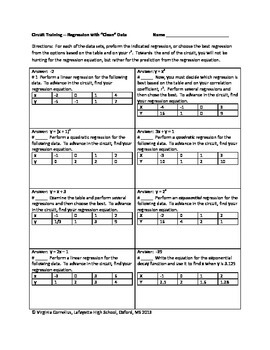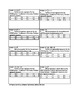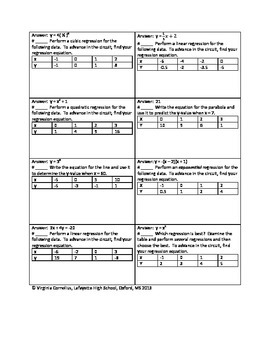# Circuit Training - Linear, Quadratic and Exponential RegressionSubject
Resource Type
File Type

PDF

(429 KB|2 pages)
Product Description

Give your students engaging practice with the circuit format! Students will need to use technology (graphing calculator, on-line software) to calculate regression equations to advance in the circuit. At first students will be told what kind of regression, but towards the end of the circuit students need to try several regressions and compare the r-value to see which is the best fit. 16 equations total. Can be turned into task cards or even a scavenger hunt.

In addition, many of the answers are algebraically equivalent (i.e. y = x^2 + 4x + 4 might be what the students get from their technology but the answer appears in factored form as y = (x + 2)^2) so as to give the students critical thinking practice with previously learned skills.

As with all of the circuits, there is no key included since the answers are embedded in the circuit. If you suspect an error or get stuck, please do not hesitate to email me at virge@virgecornelius.com.

Total Pages
2 pages
N/A
Teaching Duration
40 minutes
Report this Resource to TpT
Reported resources will be reviewed by our team. Report this resource to let us know if this resource violates TpT’s content guidelines.